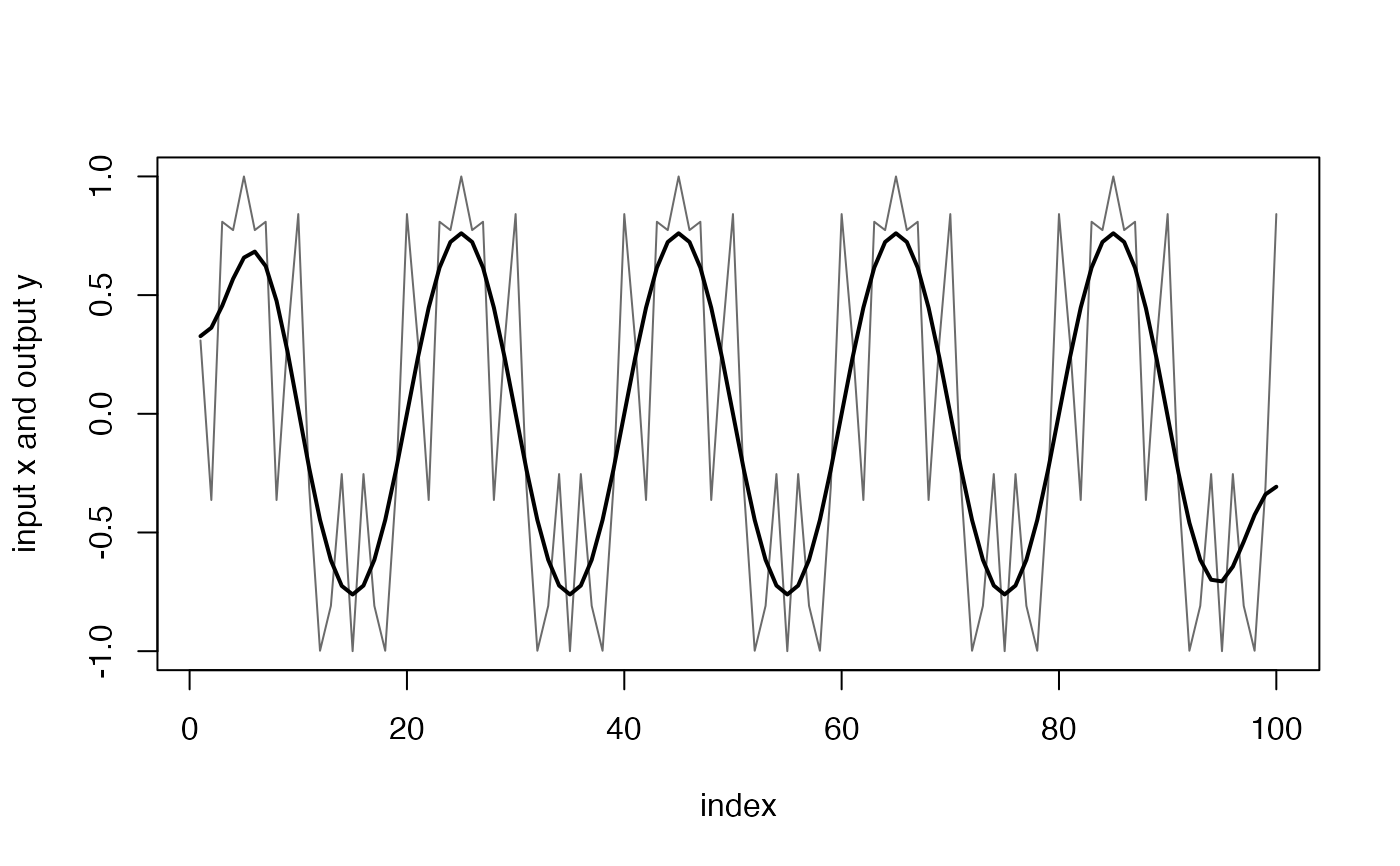This function is used to gather a delay-free filtering using a linear-phase (symmetric) FIR filter followed by group delay correction. Delay-free filtering is needed when the relative timing between signals is important e.g., when integrating signals that have been sampled at different rates.

## Usage

fir_nodelay(x, n, fc, qual = "low", return_coefs = FALSE)

## Arguments

x

The signal to be filtered. It can be multi-channel with a signal in each column, e.g., an acceleration matrix. The number of samples (i.e., the number of rows in x) must be larger than the filter length, n.

n

The length of symmetric FIR filter to use in units of input samples (i.e., samples of x). The length should be at least 4/fc. A longer filter gives a steeper cut-off.

fc

The filter cut-off frequency relative to sampling_rate/2=1. If a single number is given, the filter is a low-pass or high-pass. If fc is a vector with two numbers, the filter is a bandpass filter with lower and upper cut-off frequencies given by fc(1) and fc(2). For a bandpass filter, n should be at least 4/fc(1) or 4/diff(fc) whichever is larger.

qual

An optional qualifier determining if the filter is: "low" for low-pass (the default value if fc has a single number), or "high" for high-pass. Default is "low".

return_coefs

Logical. Return filter coefficients instead of filtered signal? If TRUE, the function will return the FIR filter coefficients instead of the filtered signal. Default is FALSE.

## Value

If return_coefs is FALSE (the default), fir_nodelay() returns the filtered signal (same size as x). If return_coefs is TRUE, returns the vector of filter coefficients only.

## Note

The filter is generated by a call to fir1: h <- fir1(n, fc, qual).

h is always an odd length filter even if n is even. This is needed to ensure that the filter is both symmetric and has a group delay which is an integer number of samples.

The filter has a support of n samples, i.e., it uses n samples from x to compute each sample in y.

The input samples used are those from n/2 samples before to n/2 samples after the sample number being computed. This means that samples at the start and end of the output vector y need input samples before the start of x and after the end of x. These are faked by reversing the first n/2 samples of x and concatenating them to the start of x. The same trick is used at the end of x. As a result, the first and last n/2 samples in y are untrustworthy. This initial condition problem is true for any filter but the FIR filter used here makes it easy to identify precisely which samples are unreliable.

## Examples

x <- sin(t(2 * pi * 0.05 * (1:100)) +
t(cos(2 * pi * 0.25 * (1:100))))
Y <- fir_nodelay(x = x, n = 30, fc = 0.2, qual = "low")
plot(c(1:length(x)), x,
type = "l", col = "grey42",
xlab = "index", ylab = "input x and output y"
)
lines(c(1:length(Y)), Y, lwd = 2)